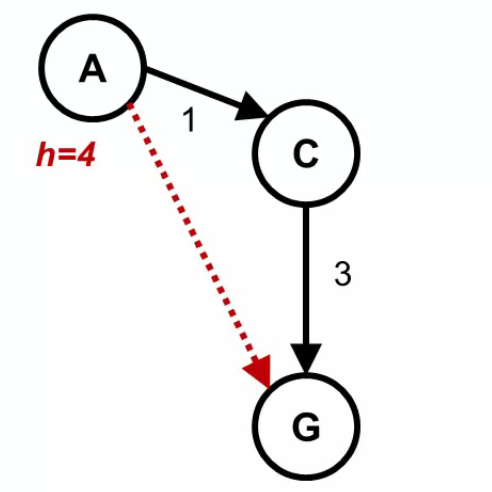2 views

In this figure:let's assume that h(C)=1 If f(A)=g(A)+h(A)=0+4=4, and f(C)=g(C)+h(C)=1+1=2 Then f(C) is NOT greater than or equal to f(A) Therefore this example is consistent and admissible, but can someone give me an example of admissible heuristic that is not consistent? please

by (108k points)

CONSISTENT HEURISTIC:

A heuristic in the algorithm is consistent if the cost from the current node to a successor node, plus the estimated cost from the successor node to the goal is less than or equal to the estimated cost from the current node to the goal.

In an equation, it would look like this: C(n, n’) + h(n’) ≤ h(n)

A heuristic function is admissible if the estimated cost is never more than the actual cost from the current node to the goal node.

If you want inconsistency and since h(C) <= 3 for the admissibility condition then you should have that h(A) > 1 + h(C). So any heristics that satisfies:

h(A) > 1 + h(C)

h(C) <= 3

h(G) = 0

is admissible and not consistent. You gave

h(A) = 4

h(C) = 1

h(G) = 0

which is a valid candidate.

If you are looking to learn more about Artificial Intelligence then you visit Artificial Intelligence Tutorial. Also, if you are appearing for job profiles of AI Engineer or AI Expert then you can prepare for the interviews on Artificial Intelligence Interview Questions.